# 0708-1300/Homework Assignment 1

(diff) ← Older revision | Latest revision (diff) | Newer revision → (diff)
Announcements go here
In Preparation

The information below is preliminary and cannot be trusted! (v)

Read sections 1-5 of chapter II of Bredon's book three times:

• First time as if you were reading a novel - quickly and without too much attention to detail, just to learn what the main keywords and concepts and goals are.
• Second time like you were studying for an exam on the subject - slowly and not skipping anything, verifying every little detail.
• And then a third time, again at a quicker pace, to remind yourself of the bigger picture all those little details are there to paint.

## Doing

• Solve and submit the following two problems:
1. Show explicitly that the restricted implicit function theorem, with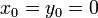$x_0=y_0=0$ and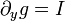$\partial_yg=I$, is equivalent to general implicit function theorem, in which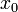$x_0$ and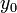$y_0$ are arbitrary and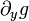$\partial_yg$ is an arbitrary invertible matrix.
2. Show that the definition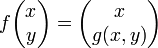$f\begin{pmatrix}x\\y\end{pmatrix}=\begin{pmatrix}x\\g(x,y)\end{pmatrix}$ reduces the implicit function theorem to the inverse function theorem. A key fact to verify is that differential of$f$ at the relevant point is invertible.
• Solve the following problems from Bredon's book, but submit only the solutions of underlined problems:
problems on page(s)
1, 2, 3, 4, 5 71
1, 2 75-76
1-4 80

## Due Date

This assignment is due in class on Thursday October 4, 2007.